# Complementary and Supplementary Angles

A pair of angles is sometimes classified based on the result of their addition as complementary and supplementary angles.

## What are Complementary Angles

Two angles are called complementary angles if the sum of their measure equals 90°. In other words, if two angles add up to form a right angle they known as complementary angles. Each angle in the pair is said to be the complement of the other.

The word ‘complementary’ came from the Latin word ‘completum’ meaning ‘completed’. To become complementary angles, the two angles do not need to be adjacent. If they are adjacent they will form a right angle.

In the above figure,

∠XOZ + ∠ZOY = 90°, and thus are complementary angels.

### How to Find Two Angles are Complementary

If the measure of two angels is given, adding them will prove whether they are complementary.

Mathematically,

Sum of two complementary angles = 90°

Thus, if one of the angle is x, the other angle will be (90° – x)

For example, in a right angle triangle, the two acute angles are complementary. This is because in a triangle the sum of the three angles is 180°. Since one angle is 90°, the sum of the other two angles forms 90°.

Let’s understand the concept using some examples: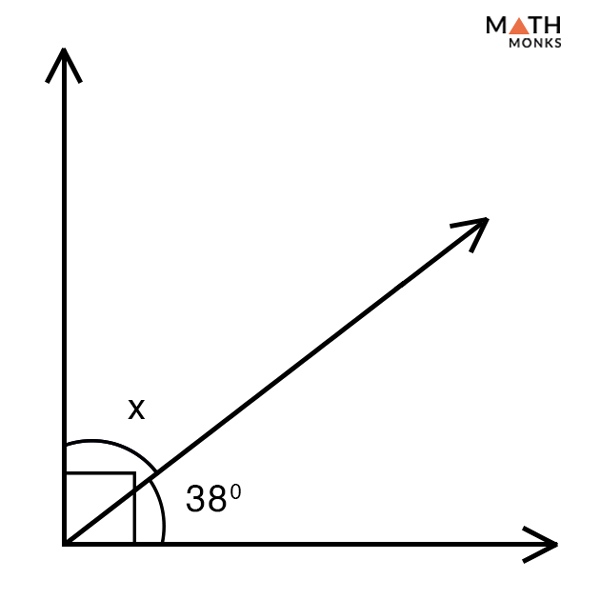Determine the missing angle.

Solution:

As we know,
Sum of two complementary angles = 90°, here one angle = 38°, other angle = x
Thus,
x + 38° = 90°
x = 90° – 38°
x = 52°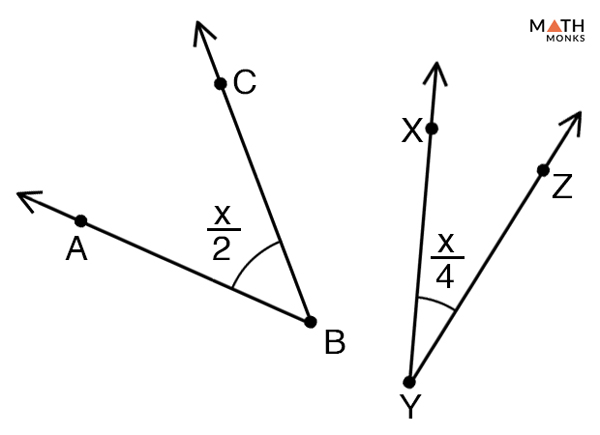Solve the value of x if the following two angles are complementary. Also find the measure of the two angles.

Solution:

As we know,
Sum of two complementary angles = 90°, here first angle = x/2, second angle = x/4
x/2 + x/4 = 90°
(2x + x)/4 = 90°
3x/4 = 90°
x = 90° x 4/3
x = 120°
Hence,
∠ABC = x/2 = 120°/2 = 60° and,
∠XYZ = x/4 = 120°/4 = 30°

If x and y are complementary angles and x = 60°. Find the value of y.

Solution:

As we know,
Sum of two complementary angles = 90°
Here,
x + y = 90°, here x = 60°
60° + y = 90°
y = 90° – 60°
y = 30°

The measure of an angle is 22°. What is the measure of its complementary angle?

Solution:

Let x be the measure of the complementary angel to 22°
As we know,
Sum of two complementary angles = 90°
x + 22° = 90°
x = 90° – 22°
x = 68°

Find the complement of the angle 1/2nd of 100°

Solution:

1/2nd of 100°
= ½ x 100°
= 50°
As we know,
Sum of two complementary angles = 90°
x + 50° = 90°
x = 90° – 50°
x = 40°

The difference between two complementary angles is 48°. Find both the angles.

Solution:

Let one of the angle be = x°
Then the other angle = (90 – x)°
Given that difference between the two angles = 48°
Now,
(90 – x)° – x° = 48°
90° – 2x = 48°
– 2x = 48° – 90°
– 2x = – 42°
x = 42°/2
x = 21°
Thus the other angle is,
(90 – x)° = 90° – 21° = 69°

Two angles are complementary. The measure of the larger angle is 10 degree more than thrice the measure of the smaller angle. Find the measure of the smaller and larger angle in degrees.

Solution:

Let us assume the two complementary angles are x and y, where x is the larger angle and y is the smaller angle.
By the given information,
x = 3y + 10…… (1)
As we know, two angles are complementary,
Hence,
x + y = 90°…… (2)
Putting the value of (1) in (2) we get,
x + y = 90° (known)
3y + 10 + y = 90° [∵x = 3y + 10]
4y = 90 – 10
y = 80/4
y = 20°
Now,
Putting the value of y in (2) we get,
x + 20° = 90°
x = 90° – 20° = 70°
Hence the larger angle (x) = 70° and the smaller angle (y) = 20°

### Types of Complementary Angles

As discussed before, two angles need not be adjacent to be complementary to each other; accordingly, they are divided into two types:

1) Adjacent Complementary Angles: Two angles are adjacent complementary angles if they share a common vertex and a common arm. An example of adjacent complementary angles is given below:

In the above figure ∠AOC and ∠BOC are adjacent angles a they have a common vertex O and a common arm OC. Also, they add up to 90°.

∠AOC + ∠BOC = 35° + 55° = 90°

Thus these two angles are adjacent complementary angles.

2) Non-adjacent Complementary Angles: Two angles are non-adjacent complementary angles they are not adjacent to each other.

Here, ∠AOB and ∠XOY are non-adjacent angles as they neither have a common vertex nor a common arm. Also, they add up to 90°.

∠AOB + ∠XOY = 35° + 55° = 90°

Thus these two angles are non-adjacent complementary angles.

### Complementary Angles Theorem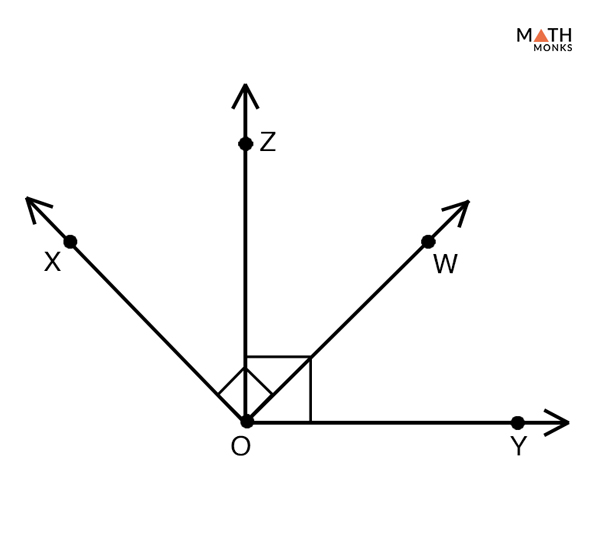Prove Complementary Angles Theorem

To prove:

The Two Angles that are Complementary to the Same Angle are Congruent to Each Other

Proof:

Let ∠ZOW is complementary to ∠XOZ and ∠YOW
Then, by the definition of complementary angles,
∠ZOW + ∠XOZ = 90°…… (1)
∠ZOW + ∠YOW = 90°…… (2)
Adding (1) and (2) we get,
∠ZOW + ∠XOZ = ∠ZOW + ∠YOW
Subtracting ∠ZOW from both sides, we get
∠XOZ = ∠YOW
Hence Proved

### Real Life Examples of Complementary Angles

• When a rectangular piece of bread is divided into two pieces by cutting along the diagonal, we get two right triangles, each with a pair of complementary angles.
• A slice of pizza
• Hands of a clock showing 3PM and the seconds hand pointing towards the digit 2
• A staircase that have an escalation of 30° and the wall at 90°

## What are Supplementary Angles

Two angles are called supplementary angles if the sum of their measure equals 180°. Each angle in the pair is said to be the supplement of the other.

The word ‘supplementary’ came from the Latin word ‘supplere’ meaning ‘supply’. Similar to complementary angles, the two angles do not need to be adjacent. If they are adjacent they will form a straight angle.

In the above figure,

∠XOZ + ∠YOZ = 180°, and thus are supplementary angels.

Thus in a pair of supplementary angles:

• If one angel is acute the other is an obtuse angle
• If one angle is 90°, the other angle is also 90°

### How to Find Two Angles are Supplementary

If the measure of two angels is given, adding them will prove whether they are supplementary.

Mathematically,

Sum of two supplementary angles = 180°

Thus, if one of the angle is x, the other angle will be (180° – x)

Let’s understand the concept using some examples: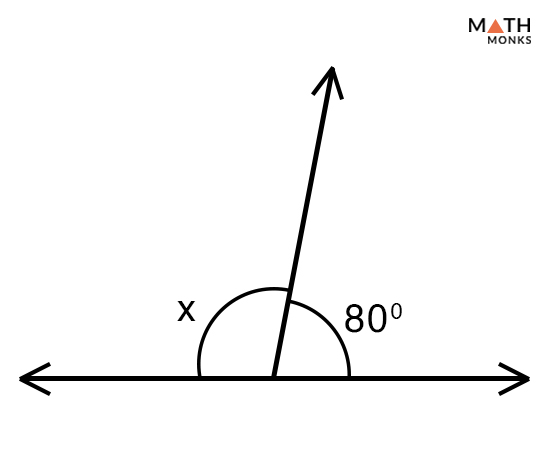Determine the missing angle.

Solution:

As we know,
Sum of two supplementary angles = 180°, here one angle = 80°, other angle = x
Thus,
x + 38° = 180°
x = 180° – 80°
x = 100°

If x and y are supplementary angles and x = 88°. Find the value of y.

Solution:

As we know,
Sum of two supplementary angles = 180°
Here,
x + y = 180°, here x = 88°
88° + y = 180°
y = 180° – 88°
y = 92°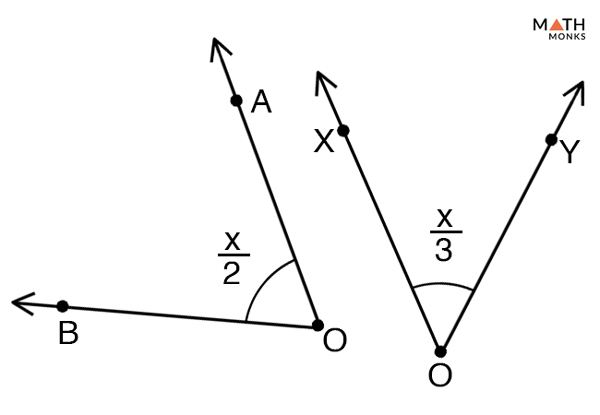Solve the value of x if the following two angles are supplementary. Also find the measure of the two angles.

Solution:

As we know,
Sum of two supplementary angles = 180°, here first angle = x/2, second angle = x/3
x/2 + x/3 = 180°
(3x + 2x)/6 = 180°
5x/6 = 180°
x = 180° x 6/5
x = 216°
Hence,
∠AOB = x/2 = 216°/2 = 108° and,
∠XOY = x/3 = 216°/3 = 72°

The measure of an angle is 120°. What is the measure of its supplementary angle?

Solution:

Let x be the measure of the supplementary angel to 120°
As we know,
Sum of two supplementary angles = 180°, here x and 120° are supplementary angles
x + 120° = 180°
x = 180° – 120°
x = 60°

Find the supplement of the angle 1/3rd of 330°

Solution:

1/3rd of 330°
= 1/3 x 330°
= 110°
As we know,
Sum of two supplementary angles = 180°
Let x be the supplement of 110°
x + 110° = 180°
x = 180° – 110°
x = 70°

The difference between two supplementary angles is 70°. Find both the angles.

Solution:

Let one of the angle be = x°
Then the other angle = (180 – x)°
Given that difference between the two angles = 70°
Now,
(180 – x)° – x° = 70°
180° – 2x = 70°
– 2x = 70° – 180°
– 2x = – 110°
x = 110°/2
x = 55°
Thus the other angle is,
(180 – x)° = 180° – 55° = 125°

Two angles are supplementary. The measure of the larger angle is 6 degree more than twice the measure of the smaller angle. Find the measure of the smaller and larger angle in degrees.

Solution:

Let us assume the two supplementary angles are x and y, where x is the larger angle and y is the smaller angle.
By the given information,
x = 2y + 6…… (1)
As we know, two angles are supplementary,
Hence,
x + y = 180°…… (2)
Putting the value of (1) in (2) we get,
x + y = 180° (known)
2y + 6+ y = 180° [∵x = 2y + 6]
3y = 180 – 6
y = 174/3
y = 58°
Now,
Putting the value of y in (2) we get,
x + 58° = 180°
x = 180° – 58° = 122°
Hence the larger angle (x) = 122° and the smaller angle (y) = 58°

### Types of Supplementary Angles

As discussed before, two angles need not be adjacent to be supplementary to each other; accordingly, they are divided into two types:

1) Adjacent Supplementary Angles: Two angles are adjacent supplementary angles if they share a common vertex and a common arm. An example of adjacent supplementary angles is given below:

In the above figure ∠BCA and ∠DCA are adjacent angles a they have a common vertex C and a common arm OA. Also, they add up to 180°.

∠ BCA + ∠ DCA = 60° + 120° = 180°

Thus these two angles are adjacent supplementary angles.

2) Non-adjacent Supplementary Angles: Two angles are non-adjacent supplementary angles they are not adjacent to each other.

Here, ∠AOB and ∠XOY are non-adjacent angles as they neither have a common vertex nor a common arm. Also, they add up to 180°.

∠AOB + ∠XOY = 35° + 55° = 180°

Thus these two angles are non-adjacent supplementary angles.

### Supplementary Angles Theorem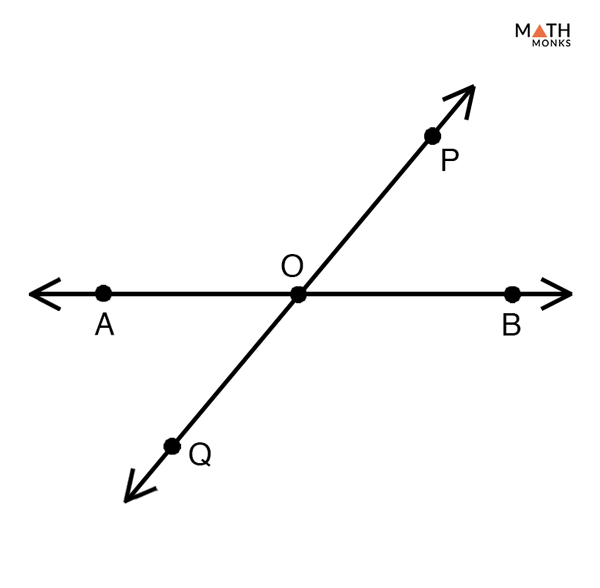Prove Supplementary Angles Theorem

To prove:

The two Angles that are Supplementary to the Same Angle are Congruent to Each Other

Proof:

Let ∠POB is supplementary to ∠AOP and ∠QOB
Then, by the definition of supplementary angles,
∠POB + ∠AOP = 180°….. (1)
∠POB + ∠QOB = 180°……(2)
Adding (1) and (2) we get,
∠POB + ∠AOP = ∠POB + ∠QOB
Subtracting ∠POB from both sides we get,
∠AOP = ∠QOB
Hence Proved

### Examples in Real Life

• Consecutive angles in a parallelogram
• Cock showing 9’ o clock and 3’o clock
• Slice of pizza
• Lines in a tennis court

## Mnemonics and Fun Facts

• The letter ‘c’ for ‘complementary’ and ‘c’ for ‘corner’. Hence when two complementary angles put together to form a ‘corner right’ angle.
• The letter‘s’ for ‘supplementary’ and‘s’ for ‘straight’. Hence when two supplementary angles are put together they form a ‘straight’ angle.
• The letter ‘c’ in complementary comes before the letter ‘s’ in supplementary, just like 90° comes before 180°.

## FAQs

Q1. Are complementary angles always congruent?

Ans. No, complementary angles are not always congruent. Since complementary angles add up to 90°, the only possible pairs of complementary angles that are congruent are they measure 45°each.

Q2. Are same side interior angles supplementary?

Ans. According to the ‘same side interior angle’ theorem if a transversal intersects two parallel lines, each pair of interior angles on the same side are supplementary

Q3. Are all supplementary angles linear pairs?

Ans. Yes, all linear pairs of angles are supplementary.